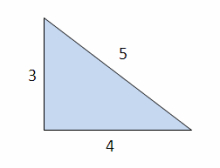# Cosine

Cosine and sine theorem:
Calculate all missing values from triangle ABC.
c = 2.9 cm; β = 28°; γ = 14° α =? °; a =? cm; b =? cm

α =  138 °
a =  8.0211 cm
b =  5.6277 cm

### Step-by-step explanation:

Try calculation via our triangle calculator.Did you find an error or inaccuracy? Feel free to write us. Thank you!

Tips for related online calculators
Do you want to convert length units?
Cosine rule uses trigonometric SAS triangle calculator.